Sunday, March 29, 2009

Regression Slope Voodoo

Anyone who is interested in the issue of global warming is immediately faced with the claim that the regression line of the temperature line shows an increase of, say for example, .5 deg / decade. The term comes under several names, such as 'trend' or 'warming per decade' etc These statistics sound so, impressive, so incontrovertable. After all, most people don't know what a regression line is.

A site that deals in statistics defines it this way:

"A regression line is a line drawn through a scatterplot of two variables. The line is chosen so that it comes as close to the points as possible." source

That actually is a more understandable definition than the one on Wikipedia found here.

Now, a regression line is wonderful if the data you are trying to represent is actually linear, that is, the the data is expected to go up in a line. But what is one to do when one is dealing in cyclical data? Linear regressions force fit a line to cyclical data every bit as much as to linear data. The problem is that fitting a line to cyclical data gives stupid results. I am going to illustrate that point in several manners.

I generated a sine wave in Excel. The data going into this sine wave are demarcated at every degree. Thus there are 360 values in my sine wave data. Below is my sin wave.Let us pretend that when the sine wave is above the x-axis, we have a warming trend and when it is below the line it is colder. By viewing this simple sine wave in this fashion we can see what stupidities the global warming hysteriacs are foisting off on the mathematically ignorant. You must be clear on the fact that this is what the global warming hysteriacs do. And then they claim that the temperature is warming or cooling based upon the slope of the linear line. In order to evaluate that claim we need to see what a regression line does with a simple sine wave. The results are below, for a simple one cycle duration.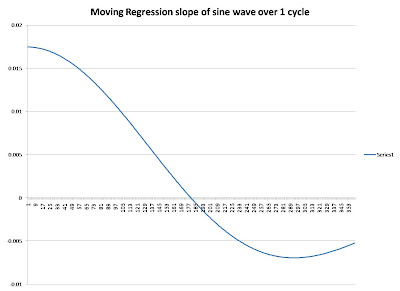This shows what is wrong with applying a linear analysis to a decidedly non-linear data stream like the temperature data. The temperature like the the sine wave, goes up and down with a complex of frequencies.

It is clear that with the sine wave that over the entire cycle, you are exactly where you were when you started. You have not gone up or down, yet, the regression slope says you are 'cooling'. If we had used -sin(x) instead of +sin(x) the regression line would have been reversed and the slope would make it appear to be warming. Yet, at the end of the sine wave you are right back where you started. So, how do we end up with a situation where the regression says we are cooling or warming when we have, in fact, ended up exactly where we were?

It lies in the mathematics of the regression curve, which I won't go deep into here, but if you remember from your High School Geometry and Algebra classes a line has a slope and an intercept. The intercept of the regression line is zero at 1 degree but never gets back to zero during the first cycle. In fact that intercept goes up to high values, higher than the sine wave can go and thus forces a negative slope on the regression, giving the appearance of a cooling or warming when in fact there is no change at all.To better illustrate the problem of representing a sinusoid with a linear regression line we will calculate the linear regression of my sinusoid over one cycle, starting at 0 degrees and going to 360 degrees. As I said, at 360 degrees the sine function is back where it started. But the regression isn't. It says that the make beleive temperature curve we have is cooling.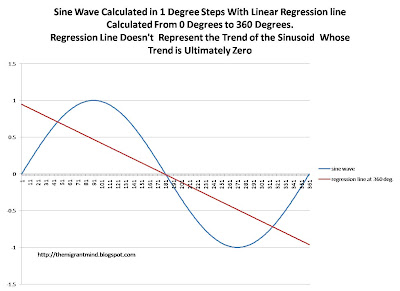We know that when it comes to climate there are sinusoids in the data which have lengths from one day's duration to hundreds of thousands of years. For many of those periodicities in the earth's climate our data, our thermometer data, doesn't even begin to cover one cycle. Yet the incompetent climatologists use the regression slope to scare us into believing that they and they alone know what the future holds. Like novice investors, they think the temperature can only go up one way.

How does the slope and regression vary over several cycles? That is interesting. See below. first the intercept then the slopeNow the slope, which wiggles around, with less amplitude than the intercept but still it shows a positive or negative trend even after 12 cycles.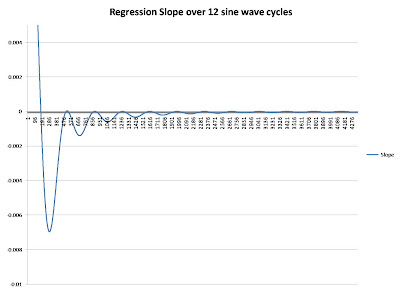We can see that the value of the 'warming' or 'cooling' depends upon how many cycles one has. With only 100 years of climate data, we don't have enough data to know what the climate is doing if the climatological cycles are longer than 100 years in duration. And we know that those cycles are longer than 100 years in duration. As documented in previous posts, the glacial cycles have durations of 19 thousand, 23, thousand, 41 thousand and 100 thousand years. Given those cycle lengths, it means that the climatologists are stupidly applying linear regression to a sinusoid with frequencies much longer duration than its data set, which is a very improper thing to do. But hey, what is science when you can scare people into giving you research money?

Now lets look at how the regression slope works for real data. It is pretty much as expected, lots of variation when the time series are short, but tending towards zero as time goes on. The chart below is for the Global temperature anomaly downloaded from NOAA's Climate at a Glance site.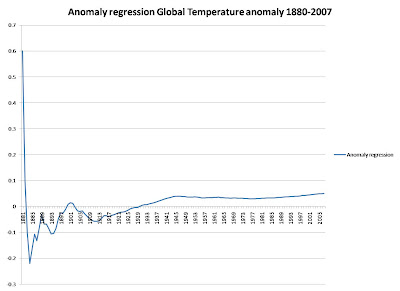The above is for 1880 to 2007. I then repeated the calculation for 1900-2007, 1920-2007, 1940-2007, 1960-2007 and 1980-2007. Then I ploted the regression slopes as a function of how long the data series is in years. You can clearly see that the slope drops as the data set becomes longer.Below is an example from Hatanga, Russia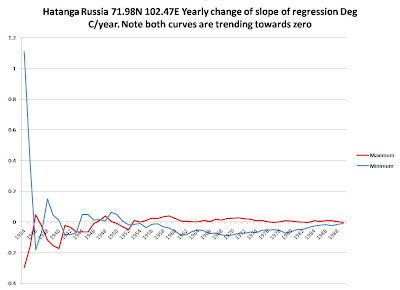Now lets look at a very long data set, the Deuterium temperature data from the Vostok ice core. It can be accessed at this site. The chart below is in deg/decade and the scale is in decades, not years, so this chart is 164,000 years long.You can see that at this scale of climatology, dealing with cycles of 100,000 years or longer, it still takes a long while for the linear regression to tend towards zero. That means that any value of the linear regression is just a number with no meaning because sinusoidal curves don't have long term up and down trends.

One final example. The regression curve on the Amundsen-Scott South Pole station says it is warming by .06 deg/decade. And the regression says that the minimum is cooling by -.3 deg/decade. Here is the plot of the data. Look at it and ask yourself if you think either of those curves is really going up or down significantly.Now lets look at the moving regression values.Clearly the longer the temperature sequence goes, the closer to zero the regression slope comes. But, since this is a linear measurement made on cyclical data, there will always be some value to the regression line. And the climatologists can use it to scare you.

You can see that the longer a sinusoidal temperature dataset goes, the lower is the slope. But, of course, linear-minded climatologists will continue to ply their linear snake-oil to the temperature data. Just remember when you hear again that the world is warming at .05 deg C/decade or some such number that you really shouldn't take it all that seriously. It is merely a mathematical artifact.

1 comment:

1.I'm so interesting the global warming issue because that's something that affect everybody in the world, I'm so scared with statistics I saw here.m10m